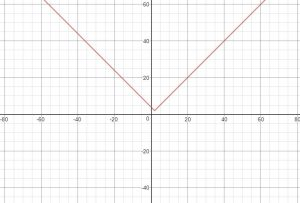# GATE | GATE CS Mock 2018 | Set 2 | Question 10

Choose the correct expression for f(x) given in the graph.(A) f(x) = 2 – |x + 2|
(B) f(x) = 2 + |x − 2|
(C) f(x) = 2 – |x − 2|
(D) f(x) = 2 + |x + 2|